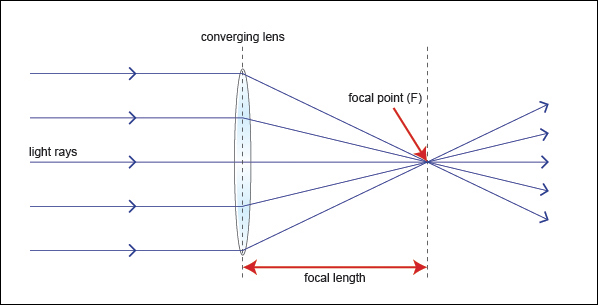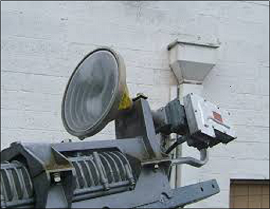# Antenna Theory - Lens

The antennas, which we have discussed till now, used the plane surface. The lens antennas use the curved surface for both transmission and reception. Lens antennas are made up of glass, where the converging and diverging properties of lens are followed. The lens antennas are used for higher frequency applications.

### Frequency Range

The frequency range of usage of lens antenna starts at 1000 MHz but its use is greater at 3000 MHz and above.

To have a better understanding of the lens antenna, the working principle of a lens has to be known. A normal glass lens works on the principle of refraction.

## Construction & Working of Lens Antenna

If a light source is assumed to be present at a focal point of a lens, which is at a focal distance from the lens, then the rays get through the lens as collimated or parallel rays on the plane wavefront.

The rays that pass through the centre of the lens are less refracted than the rays that pass through the edges of the lens. All of the rays are sent in parallel to the plane wave front. This phenomenon of lens is called as divergence.

The same procedure gets reversed if a light beam is sent from right side to left of the same lens. Then the beam gets refracted and meets at a point called focal point, at a focal distance from the lens. This phenomenon is called convergence.

The same can be better understood by observing the following diagram −The ray diagram represents the focal point and focal length from the source to the lens. The parallel rays obtained are also called as collimated rays.

In the above figure, the source at the focal point, at a focal distance from the lens, gets collimated in the plane wave front. This phenomenon can be reversed which means the light if sent from the left side, gets converged at the right side of the lens.

It is because of this reciprocity, the lens can be used as an antenna, as the same phenomenon helps in utilizing the same antenna for both transmission and reception.The image of the model of a lens antenna is shown.

To achieve the focusing properties at higher frequencies, the refractive index should be less than unity. Whatever may be the refractive index, the purpose of lens is to straighten the waveform. Based on this, the E-plane and H-plane lens are developed, which also delay or speed up the wave front.

## Types of Lens Antennas

The following types of Lens Antennas are available −

• Di-electric lens or H-plane metal plate lens or Delay lens (Travelling waves are delayed by lens media)

• E-plane metal plate lens

• Non-metallic di-electric type lens

• Metallic or artificial dielectric type of lens

The following are the advantages of Lens antenna −

• In lens antennas, feed and feed support, do not obstruct the aperture.

• It has greater design tolerance.

• Larger amount of wave, than a parabolic reflector, can be handled.

• Beam can be moved angularly with espect to the axis.

The following are the disadvantages of Lens antenna −

• Lenses are heavy and bulky, especially at lower frequencies

• Complexity in design

• Costlier compared to reflectors, for the same specifications

### Applications

The following are the applications of Lens antenna −

• Used as wide band antenna

• Especially used for Microwave frequency applications

The converging properties of lens antennas can be used for developing higher level of antennas known as Parabolic reflector antennas, which are widely used in satellite communications. We will discuss about them in the next chapter.